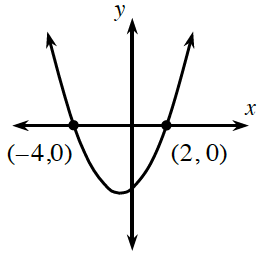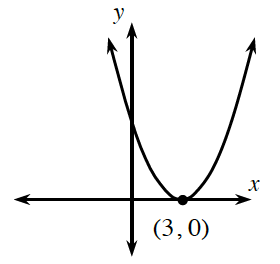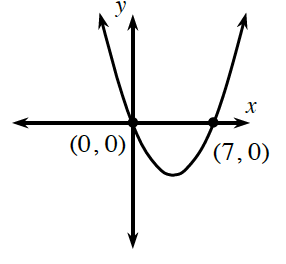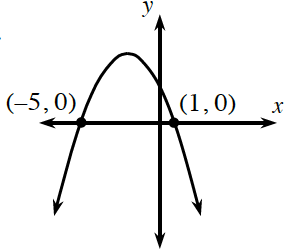### Home > CAAC > Chapter 8 > Lesson 8.2.5 > Problem8-85

8-85.

QUALITY CONTROL, Part Two

Lots O’Dough, a wealthy customer, would like to order a variety of parabolas. However, he is feeling pressed for time and said that he will pay you lots of extra money if you complete his order for him. Of course you agreed! He sent you sketches of each parabola that he would like to receive. Determine a possible equation for each parabola so that you can pass this information on to the Manufacturing Department. 8-92 HW eTool (Desmos). Homework Help ✎

1.Look at the points on this graph. What are these called?

These points are the x-intercepts of the graph.
When $−4$ or $2$ is substituted for $x$, $y$ is equal to $0$.
What could be the equation for this graph?

$y = (x + 4)(x − 2)$

1.Use the same steps found in part (a), but note that there is only one x-intercept.
What does this say about the equation?

Sometimes, the roots can be the same number.

$y=(x−3)^2\text{ or }y=x^2−6x+9$

1.1.Use the eTool below to help write an equation for each parabola.
Click on the link at right for the full eTool version: CCA 8-92 HW eTool